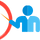# Galvanic Cell Lab

• Bid1
• Budget \$20.00
• Average Bid \$20.00

Posted on July 23, 2018

Active

#### A.Me Request Description

Materials

Galvanic cell comprised of two beakers, a salt bridge, wires and a volt meter

Electrodes: zinc, silver, copper and hydrogen

Electrolyte solutions: silver nitrate, copper (II) nitrate, zinc nitrate and hydrochloric acid.

Part 1: Pre-Lab Questions

1. Write a hypothesis about how specific electrodes and electrolytes must be combined to make a functioning cell (ex. the identiy of the anode and its electrolyte).
2. Write a hypothesis about how specific anodes and cathodes must be combined to make a functioning cell.
3. Write a hypothesis about which combination of electrodes will result in the greatest positive voltage.  As this is a galvanic cell, these are positive voltages.

REMEMBER: a hypothesis is a guess based on previous knowledge.  Try using the format IF…THEN…BECAUSE to appropriately formulate your hypotheses.  You must include the reason why you think what will happen will happen.  You may base it on previous chemistry (or physics) courses etc.

1. Given the materials above, write the half reaction for each electrode, including its Ecell value.

Part 2: The Lab

Visit:

http://www.kentchemistry.com/moviesfiles/Units/Redox/voltaiccelll20.htm

Perform enough tests using the materials provided to test your hypotheses.

Record your procedure (the electrodes and electrolyte solutions used) and your observations (the voltage produced at each test).  This can be done together in a chart type format if you like.

Part 3: Conclusions

Write a conclusion for the lab and state whether or not each hypothesis should be accepted or rejected and why.

Part 4: Post-Lab Questions

1. Explain what a negative voltage on the volt meter means.
2. Identify which wire was the anode and which was the cathode and explain how you know.
3. For each reaction that produced a positive voltage:
1. Write the balanced net ionic equation.
2. Use cell notation to describe the cell.
3. Calculate the electric potential using the standard potential table.
4. Compare your calculated values to your observed values.
5.

Chemistry

#### About the Student

5 A.Me Request(s) posted # of tutors previously hired: 1
Member since: July 22, 2018
TUTORS BIDDING (Total #: 1)
Only the student that posted the A.Me Request can view this information.
•